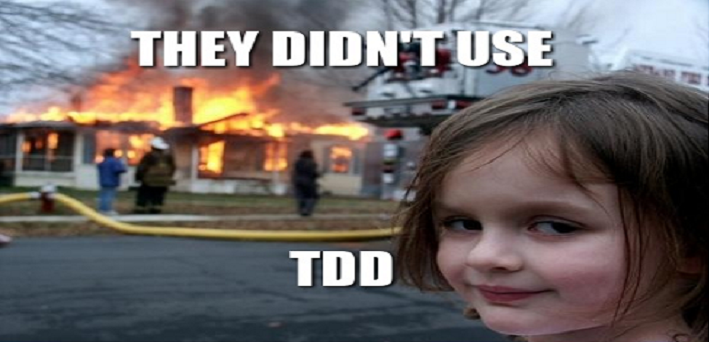# Refactoring with TDD – “Currency example”Today, I’ll show a program based on a TDD approach which I explained in a previous article. At the beginning it will have two classes representing two currencies “złoty” and “euro”. I’ll test the multiplication money and compare them like –  one euro is equal to one złoty. And every time I try to refactoring our code – remove duplications.

# Currency project

So our test for złoty looks like this:

```[Fact]
public void Multiplication_Three_Zloty_Per_Five_Result_Should_be_fifteen()
{
Zloty zloty = new Zloty(3);

var result = zloty.Multiplition(5);

Assert.Equal(15, result);
}```

Red phase is done, so try pass the test in green phase.

```public class Zloty
{
private int amount;
public Zloty(int _amount)
{
amount = _amount;
}
public float Multiplition(int value)
{
return value * amount;
}
}```

Ok. We pass our tests. At this point it’s have nothing to refactoring.

We should add the name of the currency to our class in order to later be able to recognize it more easily.

```[Fact]
public void Check_If_Name_Of_Zloty_Class_Is_PLN()
{
Zloty zloty = new Zloty(1);

Assert.Equal("PLN", zloty.currency);
}```

Test is now red so fix it.

```public class Zloty
{
public string currency;
private int amount;
public Zloty(int _amount)
{
amount = _amount;
currency = "PLN";
}
public float Multiplition(int a)
{
return a * amount;
}
}```

Now it’s everything ok.

So let’s add the Euro currency to our program.

Euro Tests:

```[Fact]
public void Multiplication_Three_Euro_Per_Five_Result_Should_be_fifteen()
{
Euro euro = new Euro(3);

var result = euro.Multiplition(5);

Assert.Equal(15, result);
}
[Fact]
public void Check_If_Name_Of_euro_Class_Is_EUR()
{
Euro euro = new Euro(1);

Assert.Equal("EUR", euro.currency);
}```

Euro Class:

```public class Euro
{
public string currency;
private int amount;
public Euro(int _amount)
{
amount = _amount;
currency = "EUR";
}
public float Multiplition(int a)
{
return a * amount;
}
}```

Hmm… ok we pass our test but… Our classes Euro and Zloty it’s almost the same. Something is wrong here, we break the principle DRY.

Writing class representing currency. Copy the whole repetitive code to class Currency and remove this code from our two classes (Zloty and Euro).

```public class Currency
{
protected int amount;
public string currency;
public Currency(int _amount)
{
amount = _amount;
}
public float Multiplition(int a)
{
return a * amount;
}
}```

Now we can refactor our tests.

```[Fact]
public void Multiplication_Three_Euro_And_Zloty_Per_Five_Result_Should_be_fifteen()
{
Currency zloty = new Zloty(3);
Currency euro = new Euro(3);

var resultPLN = zloty.Multiplition(5);
var resultEUR = euro.Multiplition(5);

Assert.Equal(15, resultPLN);
Assert.Equal(15, resultEUR);
}
[Fact]
public void Check_If_Name_Of_Zloty_Class_Is_PLN_And_Name_Of_Euro_Is_EUR()
{
Currency zloty = new Zloty(1);
Currency euro = new Euro(1);

Assert.Equal("EUR", euro.currency);
Assert.Equal("PLN", zloty.currency);
}```

We shouldn’t use two Assert on one test but it significantly reduces the length of the post. 😉

Now we have base class that represents all currency. We implement that the class of the Euro and Zloty inherits from Currency.

The question is: “Why do we need variable currency?”. It’s good question we can recognize our currency by class so now we don’t need it. Apply the principle of YANGI.

Refactor it and let’s remove test Check_If_Name_Of_Zloty_Class_Is_PLN_And_Name_Of_Euro_Is_Eur and public variable named currency.

Now let’s valid our classes.

```public class Zloty : Currency
{
public Zloty(int _amount) : base(_amount)
{
}
}

public class Euro : Currency
{
public Euro(int _amount) : base(_amount)
{
}
}```

Our tests are now positive and we remove duplication and unnecessary code. Yupi.

But at the end we should check if one euro is equals to one złoty.

Red phase:

```[Fact]
public void Check_If_One_Euro_Is_Equal_to_One_Zloty()
{
Assert.False(new Euro(1).equals(new Zloty(1)));
}
[Fact]
public void Check_If_One_Euro_Is_Equal_to_One_Euro()
{
Assert.True(new Euro(1).equals(new Euro(1)));
}```

Green phase – add method equals to Currency class. Comparing the value of the currency and whether they are of the same class.

```public bool equals(object obj)
{
Currency wal = (Currency)obj;
return amount == wal.amount && GetType().Equals(wal.GetType());
}```

After running tests, we see# Summary

We did a program that checks if the money is in the same currency. In addition with the methodology TDD thanks to our code looks nice and clear. I hope that You seen, with TDD – refactoring code is much simpler because we have larger trust that something we don’t spoil.

For homework you can write class Cantor which convert our currency 😉 . Test for it:

```[Fact]
public void Transfer_four_Zloty_Is_Equals_To_One_Euro_With_Convert_Four_Zloty_Is_Equals_To_One_Euro_Four_euro()
{
Currency zloty = new Zloty(4);

var result = Cantor.Transfer(zloty);

Assert.True(new Euro(1).equals(result));
}```

Have a nice day 😉1.Seeker says: